search
Search
Guest 0reps
Thanks for the thanks!
close
Cancel
Post
account_circle
Profile
exit_to_app
Sign out
search
keyboard_voice
close
Searching Tips
Search for a recipe:
"Creating a table in MySQL"
Search for an API documentation: "@append"
Search for code: "!dataframe"
Apply a tag filter: "#python"
Useful Shortcuts
/ to open search panel
Esc to close search panel
to navigate between search results
d to clear all current filters
Enter to expand content previewDoc SearchCode Search BetaSORRY NOTHING FOUND!
mic
Start speaking...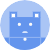Voice search is only supported in Safari and Chrome.
Shrink
Navigate to
A
A
brightness_medium
share
arrow_backShareTwitterFacebook

# Comprehensive Guide on Projections in Linear Algebra

Linear Algebra
schedule Jul 22, 2022
Last updated
local_offer Linear Algebra
Tags

# What is an orthogonal projection?

Consider the following setup: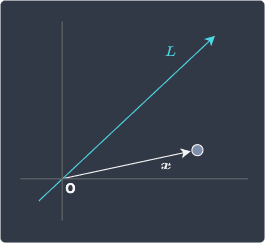Here, we have a point whose position is represented by the position vector $\boldsymbol{x}$. The shortest path from this point to the line $L$ is one that intersects the line perpendicularly: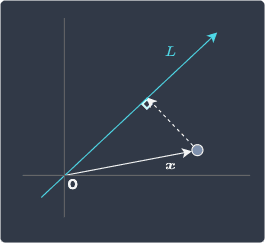The point at which this shortest path lands on line $L$ is called the projected point of $\boldsymbol{x}$ onto $L$. This point can be represented by a position vector, which we often denote as $\mathrm{Proj}_L(\boldsymbol{x})$: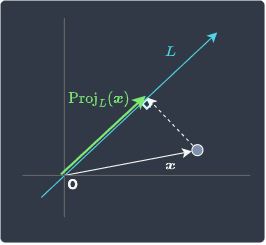We can read $\mathrm{Proj}_L(\boldsymbol{x})$ as the "orthogonal projection of position vector $\boldsymbol{x}$ onto line $L$".

Note that:

• orthogonal is a fancy way of saying perpendicular in linear algebra,

• sometimes the orthogonal part is implicit - projections in general should be interpreted as orthogonal projections.

Theorem.

# Projection of a point vector onto a line

Consider the following setup: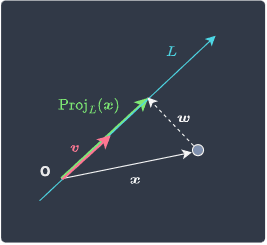Where:

• $\mathrm{Proj}_L(\boldsymbol{x})$ is the orthogonal projection of position vector $\boldsymbol{x}$ onto line $L$.

• $\boldsymbol{x}$ is the position vector that you wish to perform a projection on.

• $\boldsymbol{v}$ is any vector that points to the direction of the line $L$.

Note that we've omitted the axis lines to avoid clutter in the diagram.

To obtain the projection of a vector $\boldsymbol{x}$ onto a line $L$:

$$\mathrm{Proj}_L(\boldsymbol{x}) = \frac{\boldsymbol{v}\cdot{\boldsymbol{x}}}{\boldsymbol{v}\cdot\boldsymbol{v}} \boldsymbol{v} = \frac{\boldsymbol{v}\cdot{\boldsymbol{x}}}{\Vert\boldsymbol{v}\Vert^2} \boldsymbol{v}$$

Proof. Since vectors $\boldsymbol{v}$ and $\mathrm{Proj}_L(\boldsymbol{x})$ point in the same direction, we can express $\mathrm{Proj}_L(\boldsymbol{x})$ as some scalar multiple of $\boldsymbol{v}$:

$$\begin{equation}\label{eq:qkx0HLdtiEr2G1uPirG} \mathrm{Proj}_L(\boldsymbol{x}) = c\boldsymbol{v} \end{equation}$$

Where $c\in\mathbb{R}$ is some scalar.

Let $\boldsymbol{w}$ be the vector that points from $\boldsymbol{x}$ to $\mathrm{Proj}_L(\boldsymbol{x})$:From elementary linear algebra, we know that $\boldsymbol{w}$ can be represented like so:

$$\boldsymbol{w}=\boldsymbol{x}-\mathrm{Proj}_L(\boldsymbol{x})$$

Substituting \eqref{eq:qkx0HLdtiEr2G1uPirG} into the above yields:

\begin{align*} \boldsymbol{w}&=\boldsymbol{x}-\mathrm{Proj}_L(\boldsymbol{x})\\ \boldsymbol{w}&=\boldsymbol{x}-c\boldsymbol{v}\\ \boldsymbol{v}\cdot{\boldsymbol{w}}&= \boldsymbol{v}\cdot(\boldsymbol{x}-c\boldsymbol{v})\\ \boldsymbol{v}\cdot{\boldsymbol{w}}&= \boldsymbol{v}\cdot\boldsymbol{x}-c(\boldsymbol{v}\cdot\boldsymbol{v})\\ \end{align*}

Since $\boldsymbol{v}$ and $\boldsymbol{w}$ are orthogonal (perpendicular) to each other, their dot product is equal to the zero vector:

\begin{align*} \boldsymbol{0}&= \boldsymbol{v}\cdot\boldsymbol{x}-c(\boldsymbol{v}\cdot\boldsymbol{v})\\ c(\boldsymbol{v}\cdot\boldsymbol{v})&= \boldsymbol{v}\cdot\boldsymbol{x}\\ c&= \frac{\boldsymbol{v}\cdot\boldsymbol{x}}{\boldsymbol{v}\cdot\boldsymbol{v}}\\ \end{align*}

Substituting this result back into \eqref{eq:qkx0HLdtiEr2G1uPirG} gives:

$$\mathrm{Proj}_L(\boldsymbol{x}) = \frac{\boldsymbol{v}\cdot{\boldsymbol{x}}}{\boldsymbol{v}\cdot\boldsymbol{v}} \boldsymbol{v} = \frac{\boldsymbol{v}\cdot{\boldsymbol{x}}}{\Vert\boldsymbol{v}\Vert^2} \boldsymbol{v}$$

The second equality follows because the dot product between a vector and itself gives you the square of the vector's magnitude.

Example.

## Computing orthogonal projection

Suppose $\boldsymbol{v}$ is a vector along some line $L$:

$$\boldsymbol{v}=\begin{pmatrix} 3\\ 4\\ \end{pmatrix}$$

Let $\boldsymbol{x}$ be a position vector defined as:

$$\boldsymbol{x} =\begin{pmatrix} 1\\ 5\\ \end{pmatrix}$$

Compute the orthogonal projection of $\boldsymbol{x}$ onto line $L$.

We can directly apply our theoremlink here:

$$\begin{equation}\label{eq:KSbBa4ahL8R0FfTKtE7} \mathrm{Proj}_L(\boldsymbol{x}) = \frac{\boldsymbol{v}\cdot{\boldsymbol{x}}}{\boldsymbol{v}\cdot\boldsymbol{v}} \boldsymbol{v} \end{equation}$$

Computing the numerator:

\begin{align*} \boldsymbol{v}\cdot\boldsymbol{x}&= \begin{pmatrix} 3\\ 4\\ \end{pmatrix} \cdot\begin{pmatrix} 1\\ 5\\ \end{pmatrix}\\ &=(3)(1)+(4)(5)\\ &=23 \end{align*}

Computing the denominator:

\begin{align*} \boldsymbol{v}\cdot\boldsymbol{v}&= \begin{pmatrix} 3\\ 4\\ \end{pmatrix} \cdot\begin{pmatrix} 3\\ 4\\ \end{pmatrix}\\ &=(3)(3)+(4)(4)\\ &=25 \end{align*}

Substituting the numerator and denominator back into \eqref{eq:KSbBa4ahL8R0FfTKtE7} gives us the orthogonal projection of $\boldsymbol{x}$ onto line $L$:

$$\mathrm{Proj}_L(\boldsymbol{x}) = \frac{23}{25} \begin{pmatrix} 3\\ 4\\ \end{pmatrix}$$
Theorem.

# Projection of a point vector onto a line that is represented by a unit vector

Consider the following setup: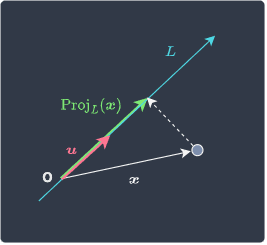Where:

• $\mathrm{Proj}_L(\boldsymbol{x})$ is the orthogonal projection of position vector $\boldsymbol{x}$ onto line $L$.

• $\boldsymbol{x}$ is the position vector that you wish to perform a projection on.

• $\boldsymbol{u}$ is a unit vector that points to the direction of the line $L$.

The only difference between this setup and the setup abovelink is that we have a unit vector $\boldsymbol{u}$ that points in the same direction as line $L$ instead of any vector $\boldsymbol{v}$.

The projection of a position vector $\boldsymbol{x}$ onto a line $L$ is computed by:

$$\mathrm{Proj}_L(\boldsymbol{x}) =(\boldsymbol{u}\cdot{\boldsymbol{x}}){\boldsymbol{u}}$$

Proof. From the previous theoremlink, we know that the projection of a vector $\boldsymbol{x}$ onto a line $L$ can be computed by:

$$\begin{equation}\label{eq:KyaOOoixxi7r0E7Fxd3} \mathrm{Proj}_L(\boldsymbol{x}) = \frac{\boldsymbol{v}\cdot{\boldsymbol{x}}}{\Vert\boldsymbol{v}\Vert^2} \boldsymbol{v} \end{equation}$$

Here, $\boldsymbol{v}$ is any vector that points to the direction of $L$.

When $\boldsymbol{v}$ is a unit vector, say $\boldsymbol{u}$, equation \eqref{eq:KyaOOoixxi7r0E7Fxd3} becomes:

\begin{align*} \mathrm{Proj}_L(\boldsymbol{x}) &=\frac{\boldsymbol{u}\cdot{\boldsymbol{x}}}{\Vert\boldsymbol{u}\Vert^2} \boldsymbol{u}\\ &=(\boldsymbol{u}\cdot{\boldsymbol{x}}){\boldsymbol{u}} \end{align*}

The equality follows because the magnitude of a unit vector is one, that is, $\Vert\boldsymbol{u}\Vert=1$.

Example.

## Computing orthogonal projections given a unit vector

Suppose $\boldsymbol{u}$ is a unit vector along some line $L$:

$$\boldsymbol{u}=\begin{pmatrix} \frac{1}{2}\\ \frac{\sqrt{3}}{2}\\ \end{pmatrix}$$

Let $\boldsymbol{x}$ be a position vector defined as:

$$\boldsymbol{x} =\begin{pmatrix} 1\\ 2\\ \end{pmatrix}$$

Compute the orthogonal projection of $\boldsymbol{x}$ onto line $L$.

We directly apply our theorem:

\begin{align*} \mathrm{Proj}_L(\boldsymbol{x}) &=(\boldsymbol{u}\cdot{\boldsymbol{x}}){\boldsymbol{u}}\\ &=\Big(\begin{pmatrix} \frac{1}{2}\\ \frac{\sqrt{3}}{2}\\ \end{pmatrix}\cdot{ \begin{pmatrix} 1\\ 2\\ \end{pmatrix}}\Big){\begin{pmatrix} \frac{1}{2}\\ \frac{\sqrt{3}}{2}\\ \end{pmatrix}}\\ &=\Big[\Big(\frac{1}{2}\Big)(1)+\Big(\frac{\sqrt{3}}{2}\Big)(2)\Big]\begin{pmatrix} \frac{1}{2}\\ \frac{\sqrt{3}}{2} \end{pmatrix}\\ &=\frac{1+2\sqrt{3}}{2} \begin{pmatrix} \frac{1}{2}\\ \frac{\sqrt{3}}{2} \end{pmatrix} \end{align*}
Theorem.

# Length of projection vector

Consider the same setup as before:The length (or the magnitude) of the projection of position vector $\boldsymbol{x}$ onto line $L$ is given by:

$$\Vert\mathrm{Proj}_L(\boldsymbol{x})\Vert =\boldsymbol{u}\cdot{\boldsymbol{x}}$$

Here, $\boldsymbol{u}$ is a unit vector that points in the same direction as $L$.

Proof. Recall from this theorem that the projection of position vector $\boldsymbol{x}$ onto line $L$ is given by:

$$\mathrm{Proj}_L(\boldsymbol{x}) =(\boldsymbol{u}\cdot{\boldsymbol{x}}){\boldsymbol{u}}$$

Here, $\boldsymbol{u}$ is a unit vector that points in the same direction as line $L$.

Let's directly take the magnitude of the projection vector:

\begin{align*} \Vert\mathrm{Proj}_L(\boldsymbol{x})\Vert &=\Vert(\boldsymbol{u}\cdot{\boldsymbol{x}}){\boldsymbol{u}}\Vert\\ &=(\boldsymbol{u}\cdot{\boldsymbol{x}})\Vert{\boldsymbol{u}}\Vert\\ &=\boldsymbol{u}\cdot{\boldsymbol{x}} \end{align*}

Here, note the following:

• the dot product $(\boldsymbol{u}\cdot{\boldsymbol{x}})$ is some scalar value, so we can take this component out of the magnitude.

• the magnitude of the unit vector $\boldsymbol{u}$ is, by definition, one.

# Application of orthogonal projection in machine learning

In machine learning, the notion of orthogonal projections comes up in dimensionality reduction techniques such as principal component analysis and linear discriminant analysis. The basic idea is to compress high-dimensional data points into lower-dimensional data points by performing orthogonal projections on them.

For instance, we can project two-dimensional data points onto a line to obtain one-dimensional points: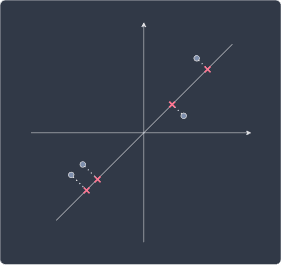Please check out our comprehensive guide on principal component analysis for the details!

mail